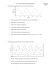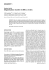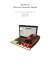# 1. A sample has a T

## Transcription

1. A sample has a T
```1. A sample has a T1 of 1.0 seconds. If the net magnetization is set equal to zero, how long
will it take for the net magnetization to recover to 98% of its equilibrium value?
2.
A sample has a T2 of 100 ms. How long will it take for any transverse magnetization to
decay to 37% of its starting value?
3. A hydrogen sample is at equilibrium in a 1.5 Tesla magnetic field. A constant B1 field of
1.17x10-4 Tesla is applied along the +x'-axis for 50 microseconds. What is the direction
of the net magnetization vector after the B1 field is turned off? Estimate the spin-lattice
relaxation time constant based on the following plot of Mz(t).
4. Sketch the frequency and phase-encoding gradient pulse waveforms necessary to generate the
following “single-shot” k-space trajectory. Parts (A) and (B) are used in the so-called spiral and
echo-planar imaging, respectively.
5.
Consider a MRI session. Assume the static field strength is B_0=1.5Tesla, the z-gradient
is Gz=2 gauss/mm, and the gyromagnetic ratio is γ =4.258 kHz/gauss. We would like to image a
slice with center position z =10cm, thickness ∆z =1mm. (a) Find the frequency range of the RF
waveform required to excite this slice and sketch the desired spectrum of the RF waveform. (b)
Write down the mathematical expression for the “ideal” waveform that will yield the desired
spectrum. (c) determine the amplitude A of this RF waveform required to induce a flip angle of
π/2.
6. Complete the table below to show what choice of TE/TR correspond to T1-weighted, T2
weighted, or Proton-Density-weighted contrast (one of the choice is not useful), in the case of a
6.
Consider an MRI scan using a pulse sequence shown below. There are M readouts total in
each slice, and you alternate the direction of the X-gradient between readouts. During each
readout duration Ts , N samples are taken. The scan parameters are chosen so that
γG yTs / 2 = γG yTy M / 2 . (a) Sketch the corresponding sampling pattern in the Frequency domain.
Indicate how the positions of the samples are related to the scan sequence parameters including
Gx , G y , Ts , Ty , M , N . (b) (10 pt) If you were to reconstruct the image using inverse 2-D discrete
Fourier transform, and you would like to reconstruct an image with M1 rows and N1 columns.
How should you choose M and N in your scan sequence? If you would like to have a pixel
resolution of ( ∆x, ∆y ), what are the constraints on the scan parameters? (i.e. give the equations
that the parameters Gx , G y , Ts , Ty , M , N must satisfy). If you want to cover an image area with size
FOVx × FOV y ,
what are the constraints on the scan parameters?
7. A fast spin-echo (FSE or RARE) sequence employs a series of 180° RF refocusing pulses to
generate multiple echoes from each excitation. Consider the diagram below showing a single
repetition of a sequence that acquires 4 echoes for each excitation
Sketch the corresponding k-space trajectory, using “×” to mark the sampling points. Be
sure to label the order of the sampling.
```

### Smart watch. Convenient as a phone stick with you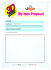### How to enter and use scan to HDD addresses onto... Bizhub Std. internal print controller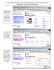### BioScanner Flyer 6.qxd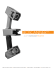### The Most Advanced Electronic Highlighter The IRISPen™ Executive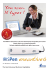### TASKalfa 4820w - KYOCERA Document Solutions America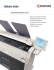### This Manual is provided FREE OF CHARGE from CBTricks.com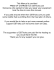### TRANS AP OUR SPECIAL SAUCE, WHY WE ARE BETTER NO SOFTWARE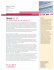### Guide to scanning in eClinical Works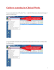### LAH IMG Order Form - ORTHO - CFO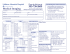### TASKalfa 2420w - KYOCERA Document Solutions America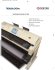### 1. The following questions refer to the waveform shown below. a